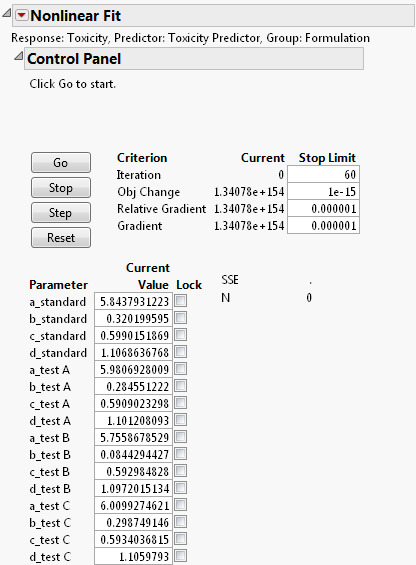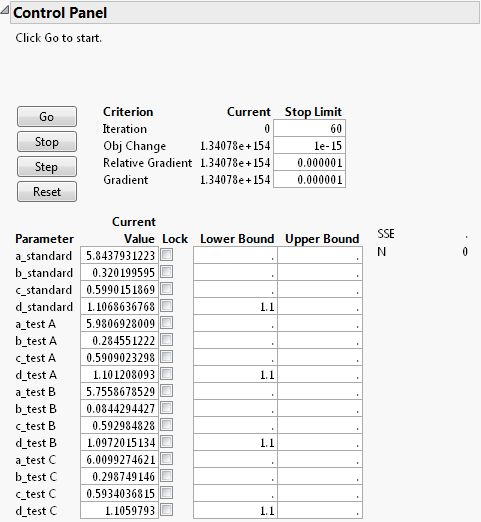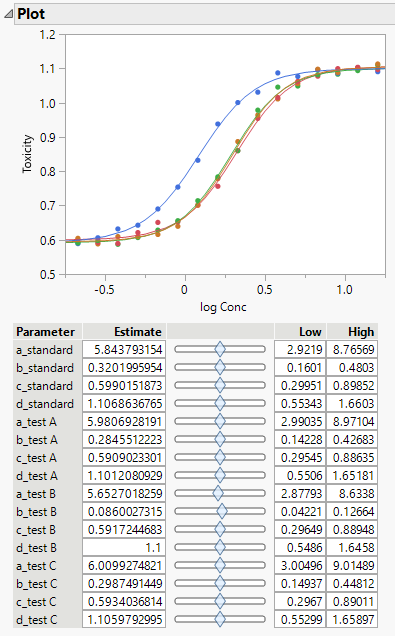Publication date: 11/10/2021

## Example of Setting Parameter Limits

The Fit Curve personality enables you to fit a model and then use the prediction equation in the full personality of the Nonlinear platform. This method requires more steps and user input but allows any nonlinear model to be fit.

Complete Example Using the Fit Curve Platform to fit the model. This example shows how to save the prediction formula from Fit Curve and then set parameter limits in Nonlinear.

1. Click the Logistic 4P red triangle and select Save Formulas > Save Parametric Prediction Formula.

A new column named Toxicity Predictor appears in the data table.

2. Select Analyze > Specialized Modeling > Nonlinear.

3. Assign Toxicity to the Y, Response role.

4. Assign Toxicity Predictor to the X, Predictor Formula role.

5. Assign Formulation to the Group role.

6. Click OK.

The Nonlinear Fit window appears. In the Control Panel, parameter values and locking options are shown. The letters listed before each parameter correspond to variables from the Prediction Model in the Fit Curve function.

Figure 15.18 Nonlinear Fit Control PanelTip: You can lock parameters if you know the values from prior information.

7. Click the Nonlinear Fit red triangle and select Parameter Bounds.

Options for setting the lower and upper parameters appear next to the parameters.

8. Set the lower bounds for the parameters as shown in Figure 15.19. You know from prior experience that the maximum toxicity of the drug is at least 1.1.

Figure 15.19 Setting Parameter Bounds9. Click Go.

The final parameter estimates are shown in the Solution report, along with other fit statistics. The fitted model is shown on the plot.

Figure 15.20 Nonlinear Fit Plot and Parameter EstimatesOptions below the plot allow for adjusting parameter limits and estimates.